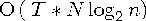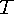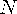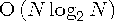The MEANS Procedure

# OUTPUT Statement

Writes statistics to a new SAS data set.
 Tip: You can use multiple OUTPUT statements to create several OUT= data sets. Featured in:

 OUTPUT ;

OUT=SAS-data-set

names the new output data set. If SAS-data-set does not exist, then PROC MEANS creates it. If you omit OUT=, then the data set is named DATAn, where n is the smallest integer that makes the name unique.

 Default: DATAn Tip: You can use data set options with the OUT= option. See Data Set Options for a list.
output-statistic-specification(s)

specifies the statistics to store in the OUT= data set and names one or more variables that contain the statistics. The form of the output-statistic-specification is

 statistic-keyword<(variable-list)>=
where
statistic-keyword

specifies which statistic to store in the output data set. The available statistic keywords are

 Descriptive statistics keyword CSS RANGE CV SKEWNESS | SKEW KURTOSIS | KURT STDDEV | STD LCLM STDERR MAX SUM MEAN SUMWGT MIN UCLM MODE USS N VAR NMISS Quantile statistics keyword MEDIAN | P50 Q3 | P75 P1 P90 P5 P95 P10 P99 Q1 | P25 QRANGE Hypothesis testing keyword PROBT | PRT T

By default the statistics in the output data set automatically inherit the analysis variable's format, informat, and label. However, statistics computed for N, NMISS, SUMWGT, USS, CSS, VAR, CV, T, PROBT, PRT, SKEWNESS, and KURTOSIS will not inherit the analysis variable's format because this format might be invalid for these statistics (for example, dollar or datetime formats).

 Restriction: If you omit variable and name(s), then PROC MEANS allows the statistic-keyword only once in a single OUTPUT statement, unless you also use the AUTONAME option. Featured in:
variable-list

specifies the names of one or more numeric analysis variables whose statistics you want to store in the output data set.

 Default: all numeric analysis variables
name(s)

specifies one or more names for the variables in output data set that will contain the analysis variable statistics. The first name contains the statistic for the first analysis variable; the second name contains the statistic for the second analysis variable; and so on.

 Default: the analysis variable name. If you specify AUTONAME, then the default is the combination of the analysis variable name and the statistic-keyword. If you use the CLASS statement and an OUTPUT statement without an output-statistic-specification, then the output data set contains five observations for each combination of class variables: the value of N, MIN, MAX, MEAN, and STD. If you use the WEIGHT statement or the WEIGHT option in the VAR statement, then the output data set also contains an observation with the sum of weights (SUMWGT) for each combination of class variables. Interaction: If you specify variable-list, then PROC MEANS uses the order in which you specify the analysis variables to store the statistics in the output data set variables. Tip: Use the AUTONAME option to have PROC MEANS generate unique names for multiple variables and statistics. Featured in: Computing Output Statistics
id-group-specification

combines the features and extends the ID statement, the IDMIN option in the PROC statement, and the MAXID and MINID options in the OUTPUT statement to create an OUT= data set that identifies multiple extreme values. The form of the id-group-specification is

 IDGROUP (> < > OUT <[n]> (id-variable-list)=)
MIN|MAX(variable-list)

specifies the selection criteria to determine the extreme values of one or more input data set variables specified in variable-list. Use MIN to determine the minimum extreme value and MAX to determine the maximum extreme value.

When you specify multiple selection variables, the ordering of observations for the selection of n extremes is done the same way that PROC SORT sorts data with multiple BY variables. PROC MEANS concatenates the variable values into a single key. The MAX(variable-list) selection criterion is similar to using PROC SORT and the DESCENDING option in the BY statement.

 Default: If you do not specify MIN or MAX, then PROC MEANS uses the observation number as the selection criterion to output observations. Restriction: If you specify criteria that are contradictory, then PROC MEANS uses only the first selection criterion. Interaction: When multiple observations contain the same extreme values in all the MIN or MAX variables, PROC MEANS uses the observation number to resolve which observation to write to the output. By default, PROC MEANS uses the first observation to resolve any ties. However, if you specify the LAST option, then PROC MEANS uses the last observation to resolve any ties.
LAST

specifies that the OUT= data set contains values from the last observation (or the last n observations, if n is specified). If you do not specify LAST, then the OUT= data set contains values from the first observation (or the first n observations, if n is specified). The OUT= data set might contain several observations because in addition to the value of the last (first) observation, the OUT= data set contains values from the last (first) observation of each subgroup level that is defined by combinations of class variable values.

 Interaction: When you specify MIN or MAX and when multiple observations contain the same extreme values, PROC MEANS uses the observation number to resolve which observation to save to the OUT= data set. If you specify LAST, then PROC MEANS uses the later observations to resolve any ties. If you do not specify LAST, then PROC MEANS uses the earlier observations to resolve any ties.
MISSING

specifies that missing values be used in selection criteria.

 Alias: MISS
OBS

includes an _OBS_ variable in the OUT= data set that contains the number of the observation in the input data set where the extreme value was found.

 Interaction: If you use WHERE processing, then the value of _OBS_ might not correspond to the location of the observation in the input data set. Interaction: If you use [n] to write multiple extreme values to the output, then PROC MEANS creates n _OBS_ variables and uses the suffix n to create the variable names, where n is a sequential integer from 1 to n.
[n]

specifies the number of extreme values for each variable in id-variable-list to include in the OUT= data set. PROC MEANS creates n new variables and uses the suffix _n to create the variable names, where n is a sequential integer from 1 to n.

By default, PROC MEANS determines one extreme value for each level of each requested type. If n is greater than one, then n extremes are output for each level of each type. When n is greater than one and you request extreme value selection, the time complexity is, whereis the number of types requested andis the number of observations in the input data set. By comparison, to group the entire data set, the time complexity is.

 Default: 1 Range: an integer between 1 and 100 Featured in: For example, to output two minimum extreme values for each variable, use `idgroup(min(x) out(x y z)=MinX MinY MinZ);`The OUT= data set contains the variables MinX_1, MinX_2, MinY_1, MinY_2, MinZ_1, and MinZ_2.
(id-variable-list)

identifies one or more input data set variables whose values PROC MEANS includes in the OUT= data set. PROC MEANS determines which observations to output by the selection criteria that you specify (MIN, MAX, and LAST).

 Alias: IDGRP Requirement: You must specify the MIN|MAX selection criteria first and OUT(id-variable-list)= after the suboptions MISSING, OBS, and LAST. Tip: You can use id-group-specification to mimic the behavior of the ID statement and a maximum-id-specification or minimum-id-specification in the OUTPUT statement. Tip: When you want the output data set to contain extreme values along with other id variables, it is more efficient to include them in the id-variable-list than to request separate statistics. For example, the statement `output idgrp(max(x) out(x a b)= );` is more efficient than the statement `output idgrp(max(x) out(a b)= ) max(x)=;` Featured in: Computing Output Statistics and Identifying the Top Three Extreme Values with the Output Statistics
name(s)

specifies one or more names for variables in the OUT= data set.

 Default: If you omit name, then PROC MEANS uses the names of variables in the id-variable-list. Tip: Use the AUTONAME option to automatically resolve naming conflicts.
CAUTION:
The IDGROUP syntax allows you to create output variables with the same name.

When this action happens, only the first variable appears in the output data set. Use the AUTONAME option to automatically resolve these naming conflicts.Note:   If you specify fewer new variable names than the combination of analysis variables and identification variables, then the remaining output variables use the corresponding names of the ID variables as soon as PROC MEANS exhausts the list of new variable names.maximum-id-specification(s)

specifies that one or more identification variables be associated with the maximum values of the analysis variables. The form of the maximum-id-specification is

 MAXID <(variable-1 <(id-variable-list-1)> <...variable-n <(id-variable-list-n)>>)> = name(s)
variable

identifies the numeric analysis variable whose maximum values PROC MEANS determines. PROC MEANS can determine several maximum values for a variable because, in addition to the overall maximum value, subgroup levels, which are defined by combinations of class variables values, also have maximum values.

 Tip: If you use an ID statement and omit variable, then PROC MEANS uses all analysis variables.
id-variable-list

identifies one or more variables whose values identify the observations with the maximum values of the analysis variable.

 Default: the ID statement variables
name(s)

specifies the names for new variables that contain the values of the identification variable associated with the maximum value of each analysis variable.

 Tip: If you use an ID statement, and omit variable and id-variable, then PROC MEANS associates all ID statement variables with each analysis variable. Thus, for each analysis variable, the number of variables that are created in the output data set equals the number of variables that you specify in the ID statement. Tip: Use the AUTONAME option to automatically resolve naming conflicts. Note:   If multiple observations contain the maximum value within a class level, then PROC MEANS saves the value of the ID variable for only the first of those observations in the output data set.Featured in: Identifying an Extreme Value with the Output Statistics
CAUTION:
The MAXID syntax allows you to create output variables with the same name.

When this action happens, only the first variable appears in the output data set. Use the AUTONAME option to automatically resolve these naming conflicts.Note:   If you specify fewer new variable names than the combination of analysis variables and identification variables, then the remaining output variables use the corresponding names of the ID variables as soon as PROC MEANS exhausts the list of new variable names.minid-specification

See the description of maximum-id-specification. This option behaves in exactly the same way, except that PROC MEANS determines the minimum values instead of the maximum values. The form of the minid-specification is

 MINID<(variable-1 <(id-variable-list-1)> <...variable-n <(id-variable-list-n)>>)> = name(s)

When MINID is used without an explicit variable list, it is similar to the following more advanced IDGROUP syntax example:

 IDGRP( min(x) missing out(id_variable)=idminx) idgrp( min(y) missing out(id_variable)=idminy)

If one or more of the analysis variables has a missing value, the id_variable value will correspond to the observation with the missing value not the observation with the value for the MIN statistic.

AUTOLABEL

specifies that PROC MEANS appends the statistic name to the end of the variable label. If an analysis variable has no label, then PROC MEANS creates a label by appending the statistic name to the analysis variable name.

AUTONAME

specifies that PROC MEANS creates a unique variable name for an output statistic when you do not assign the variable name in the OUTPUT statement. This action is accomplished by appending the statistic-keyword to the end of the input variable name from which the statistic was derived. For example, the statement

`output min(x)=/autoname;`
produces the x_Min variable in the output data set.

AUTONAME activates the SAS internal mechanism to automatically resolve conflicts in the variable names in the output data set. Duplicate variables will not generate errors. As a result, the statement

`output min(x)= min(x)=/autoname;`
produces two variables, x_Min and x_Min2, in the output data set.
KEEPLEN

specifies that statistics in the output data set inherit the length of the analysis variable that PROC MEANS uses to derive them.

CAUTION:
You permanently lose numeric precision when the length of the analysis variable causes PROC MEANS to truncate or round the value of the statistic. However, the precision of the statistic will match that of the input.LEVELS

includes a variable named _LEVEL_ in the output data set. This variable contains a value from 1 to n that indicates a unique combination of the values of class variables (the values of _TYPE_ variable).

 Main discussion: Output Data Set Featured in: Computing Output Statistics
NOINHERIT

specifies that the variables in the output data set that contain statistics do not inherit the attributes (label and format) of the analysis variables which are used to derive them.

 Interaction When no option is used (implied INHERIT) then the statistics inherit the attributes, label and format, of the input analysis variable(s). If the INHERIT option is used on the OUTPUT statement, then the statistics inherit the length of the input analysis variable(s), the label and format. Tip: By default, the output data set includes an output variable for each analysis variable and for five observations that contain N, MIN, MAX, MEAN, and STDDEV. Unless you specify NOINHERIT, this variable inherits the format of the analysis variable, which can be invalid for the N statistic (for example, datetime formats).
WAYS

includes a variable named _WAY_ in the output data set. This variable contains a value from 1 to the maximum number of class variables that indicates how many class variables PROC MEANS combines to create the TYPE value.

 Main discussion: Output Data Set See also: WAYS Statement Featured in: Computing Output StatisticsPrevious Page | Next Page | Top of Page